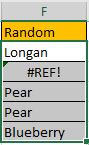# How to Generate Random Values by a List in Different Cases in Excel

Sometimes we may create some fake data for testing or some other purpose. If we want to create an amount of numbers, we may feel it is annoying by entering random numbers one by one. For this instance, we can use RANDBETWEEN function to create a lot of numbers between a certain range quickly. On the other side, sometimes we may want to create random values refer to a given list, for example select some random numbers from a given number list, you can also select objects by given list as well. This article will show you the way to generate random values, and also generate values from a given list.

## Method 1: Generate Random Numbers by RANDBETWEEN Function

Step 1: Select a range for saving the random numbers. Select a blank cell as the first cell in your selected range. Then enter the formula =RANDBETWEEN(1,100). In this case, we will generate numbers between 1 and 100.

Step 2: Press Enter. We get a random number 57. Just between our specified numbers.

Step 3: Drag the fill handle to fill the selected range. Now we create a range of random data. You may notice that the first cell value is changed. That’s because the formula is applied to other cells so after releasing your mouse to complete the task, it was recalculated.

## Method 2: Generate Random Numbers by a Given List

Prepare a list with fruit.

Step 1: Select a blank cell, enter the formula =INDEX(\$D\$2:\$D\$11, RANDBETWEEN(2, 11)). In this formula \$D\$2:\$D\$11 is the list area, 2 is the first row number for the area, and 11 is the last row number for the area. You can change parameters per your demands.

Step 2: Press Enter to check result. Verify that the random value is generated properly and it is also listed in original fruit list.

Step 3: Drag the fill handle down till F6. Verify that fruits are generated by random.

NOTICE:

1. In this step, when dragging fill handle to fill other cells, some errors may occur, for example:
2.You just need to double click on error cell and re-apply the formula.

1. Sometimes the random values may be duplicate. See above screenshot, ‘Pear’ are displayed twice.

## Method 3: Generate Random Numbers by a Given List Between Certain Range

Prepare a list with numbers. If we want to generate random values from this list and at the same time between number 1 to 50, how can we do? Let’s see steps below.

Step 1: Select a blank cell, enter the formula =INDEX(\$A\$1:\$A\$14, RANDBETWEEN(1,50)). Then press Enter to check if the generated number matches the two criteria.

Obviously, number 37 is between range 1 to 50, and it is also listed in original list.

### Related Functions

• Excel INDEX function
The Excel INDEX function returns a value from a table based on the index (row number and column number)The INDEX function is a build-in function in Microsoft Excel and it is categorized as a Lookup and Reference Function.The syntax of the INDEX function is as below:= INDEX (array, row_num,[column_num])…
• Excel RANDBETWEEN Function
The Excel RANDBETWEEN function returns a random integer number that is between the numbers you specify.The syntax of the RANDBETWEEN function is as below:= RANDBETWEEN (bottom,top)….